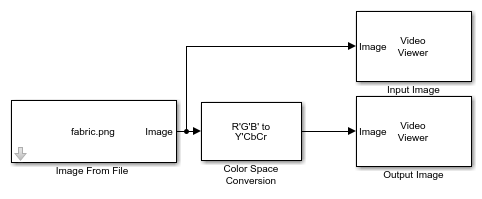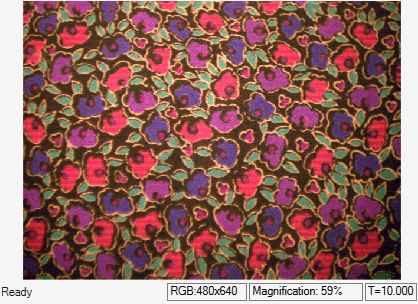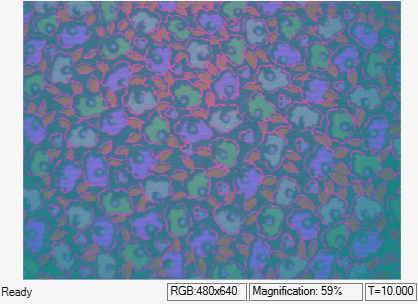# Convert Image Color Space from RGB to YCbCr

This example shows you how to convert an image color space from RGB to YCbCr.

### Example Model

```modelname = "ex_blkconvertcolorspace.slx"; open_system(modelname); ```This model reads an input image using the Image From File block, then converts the input image from the RGB color space to the YCbCr color space by using a Color Space Conversion block with these parameter values:

• Conversion`R'G'B' to Y'CbCr`

• Use conversion specified by`Rec. 601 (SDTV)`

• Image signal`One multidimensional signal`

### Simulate the Model

Run the model. The model displays the RGB color space input image and the converted YCbCr color space output image using Video Viewer blocks.

```sim(modelname); ```